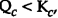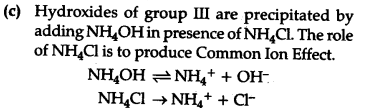# (a) The reaction quotient of a reversible reaction is Q. and the equilibrium constant is K .What do you conclude from the reaction if <Kp?

(a) The reaction quotient of a reversible reaction is Q. and the equilibrium constant is K .What do you conclude from the reaction if <Kp?
(b)State Le-Chatelier’s principle ?
©In Qualitative analysis, N\${{H}{4}}\$\${{C}{1}}\$ is added before adding N\${{H}_{4}}\$OH solution for testing of III group radicals [\${{Fe}^{3+}}\$, \${{Cr}^{3+}}\$ and \${Al}^{3+}}\$]. Explain by using common ion effect ?

(a)Ifthe reaction tends towards forward j direction to attain equilibrium.
(b)Le-Chatelier’s principle :Ifa system in equilibrium is subjected to a change of concentration,temperature or pressure, equilibrium shifts in a direction that tends to undo the effect of the change.
©Due to common Ion effect, the degree of dissoriation of N\${{H}_{4}}OH is suppressed and less OH- are formed. This less concentration of OH" is suffident to precipitate group HI cations but not the cations of higher groups since the {{K}^{sp}}\$of group III < subsequent